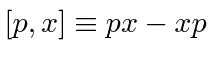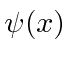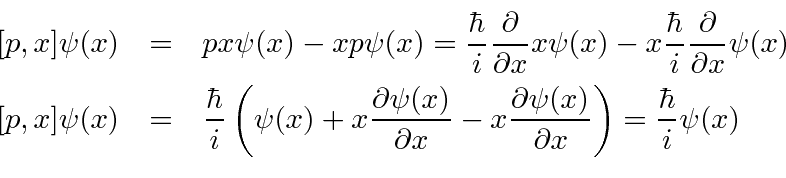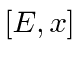## Commutators

Operators (or variables in quantum mechanics) do not necessarily commute. We can see our first example of that now that we have a few operators. We define the commutator to be(usingandas examples.)

We will now compute the commutator betweenand. Becauseis represented by a differential operator, we must do this carefully. Lets think of the commutator as a (differential) operator too, as generally it will be. To make sure that we keep all thethat we need, we will computethen remove theat the end to see only the commutator.So, removing thewe used for computational purposes, we get the commutator.Later we will learn to derive the uncertainty relation for two variables from their commutator. Physical variable with zero commutator have no uncertainty principle and we can know both of them at the same time.

We will also use commutators to solve several important problems.

We can compute the same commutator in momentum space.The commutator is the same in any representation.

* Example: Compute the commutator.*
* Example: Compute the commutator.*
* Example: Compute the commutator.*
* Example: Compute the commutator of the angular momentum operators.*

Gasiorowicz Chapter 3

Griffiths Chapter 3

Cohen-Tannoudji et al. Chapter

Jim Branson 2013-04-22